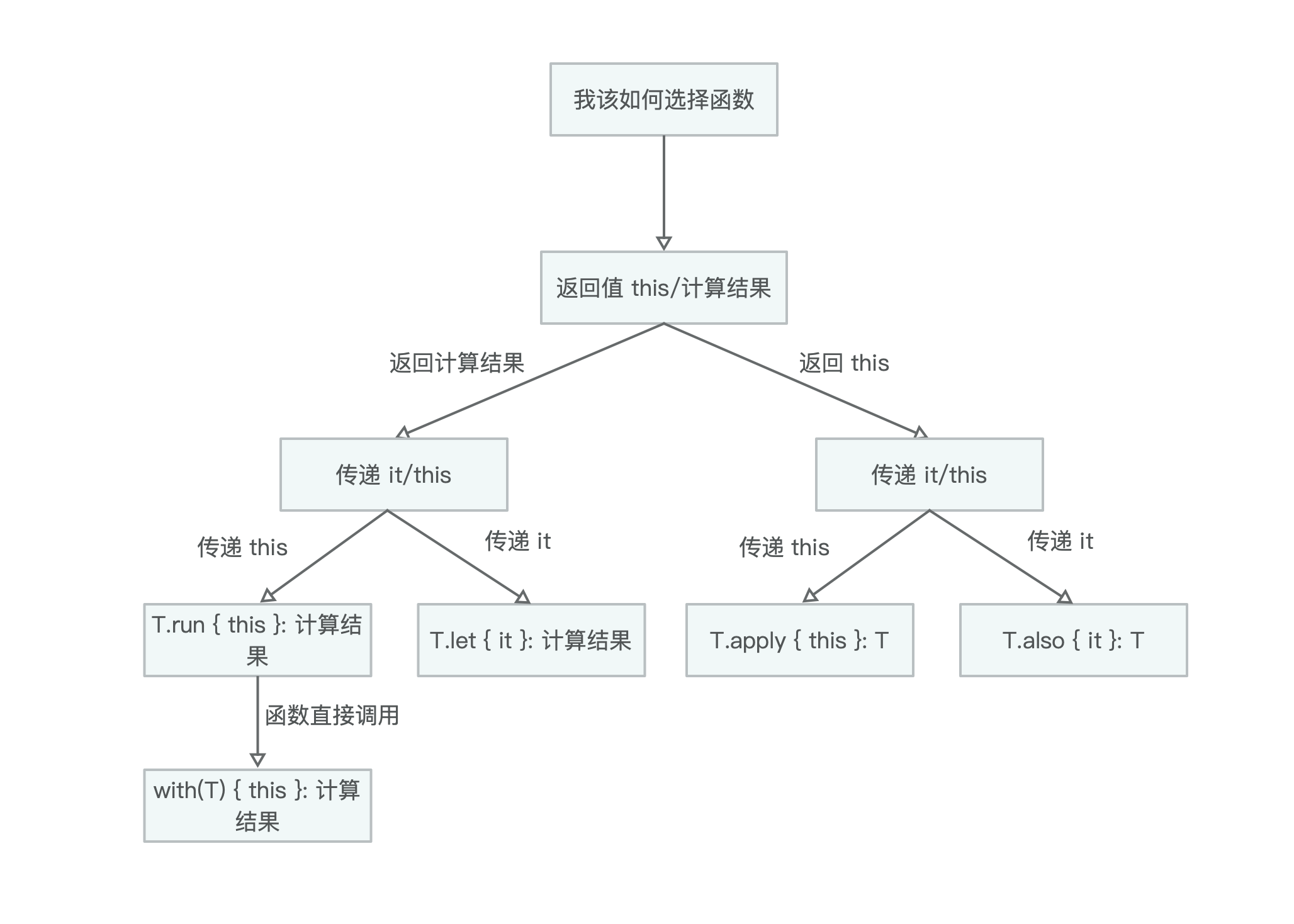Kotlin 中的 let, with, run, apply, also 等函数的使用

### apply

apply 函数和 run 函数很像，但是 apply 最后返回的是调用对象自身。

### 总结

letpublic inline fun <T, R> T.let(block: (T) -> R): R = block(this)
withpublic inline fun <T, R> with(receiver: T, block: T.() -> R): R = receiver.block()
runpublic inline fun <T, R> T.run(block: T.() -> R): R = block()
applypublic inline funT.apply(block: T.() -> Unit): T { block(); return this }
alsopublic inline fun T.also(block: (T) -> Unit): T { block(this); return this }